#### Other information models

The model given so far in this section is only one of many interesting alternatives. Suppose, for example, that the robot carries a compass that always indicates its direction. In this case, there is no need to keep track of the direction as part of the state. The robot can use the compass to specify actions directly with respect to global directions. Suppose that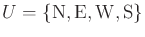, which denote the directions, north,'' east,'' west,'' and south,'' respectively. Examples 12.1 and 12.2 now become trivial. The first one is solved by applying the action sequence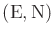. The symmetry problems vanish for Example 12.2, which can also be solved by the sequencebecause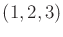is the only sequence of positions that is consistent with the actions and compass readings.

Other interesting models can be made by giving the robot less information. In the models so far, the robot can easily infer its current position relative to its starting position. Even though it is not necessarily known where this starting position lies on the map, it can always be expressed in relative coordinates. This is because the robot relies on different forms of odometry. For example, if the direction is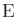and the robot executes the sequence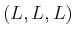, it is known that the direction is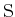because three lefts make a right. Suppose that instead of a grid, the robot must explore a graph. It moves discretely from vertex to vertex by applying an action that traverses an edge. Let this be a planar graph that is embedded in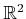and is drawn with straight line segments. The number of available actions can vary at each vertex. We can generally define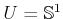, with the behavior that the robot only rotates without translating whenever a particular direction is blocked (this is a generalization of the grid case). A sensor can be defined that indicates which actions will lead to translations from the current vertex. In this case, the model nicely generalizes the original model for the grid. If the robot knows the angles between the edges that arrive at a vertex, then it can use angular odometry to make a local coordinate system inthat keeps track of its relative positions.

The situation can be made very confusing for the robot. Suppose that instead of, the action set at each vertex indicates which edges can be traversed. The robot can traverse an edge by applying an action, but it does not know anything about the direction relative to other edges. In this case, angular odometry can no longer be used. It could not, for example, tell the difference between traversing a rhombus, trapezoid, or a rectangle. If angular odometry is possible, then some symmetries can be avoided by noting the angles between the edges at each vertex. However, the new model does not allow this. All vertices that have the same degree would appear identical.

Steven M LaValle 2020-08-14